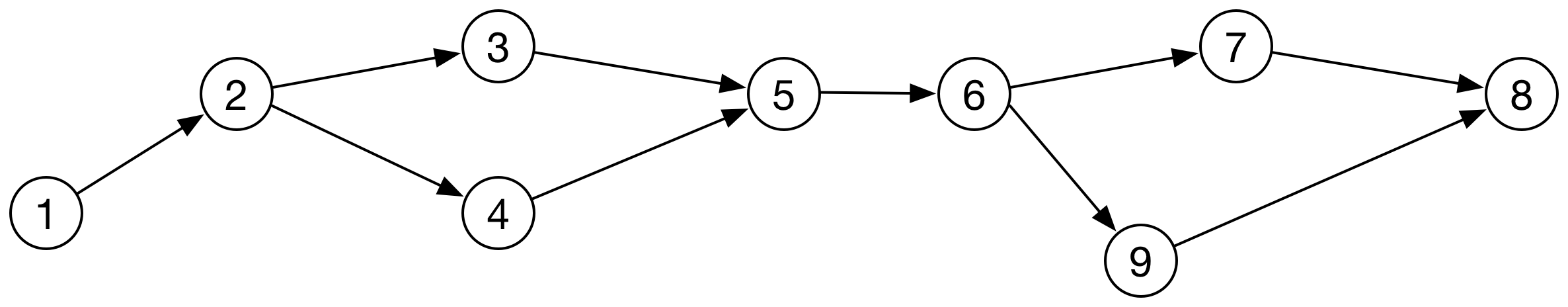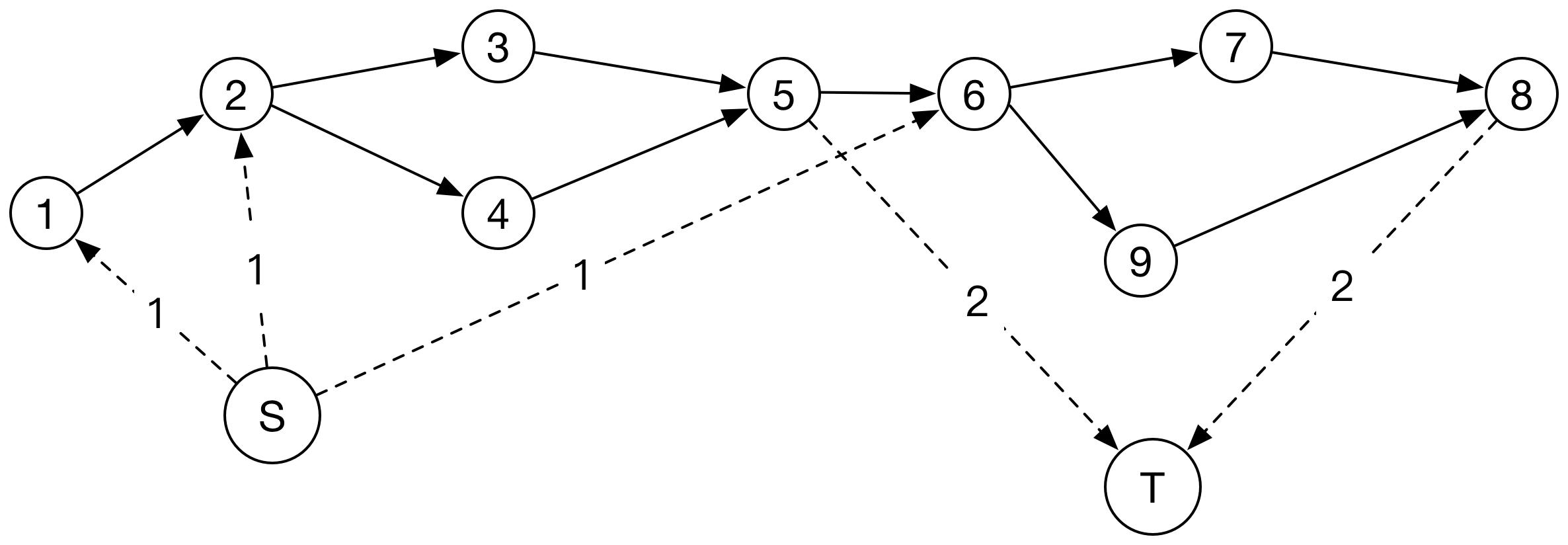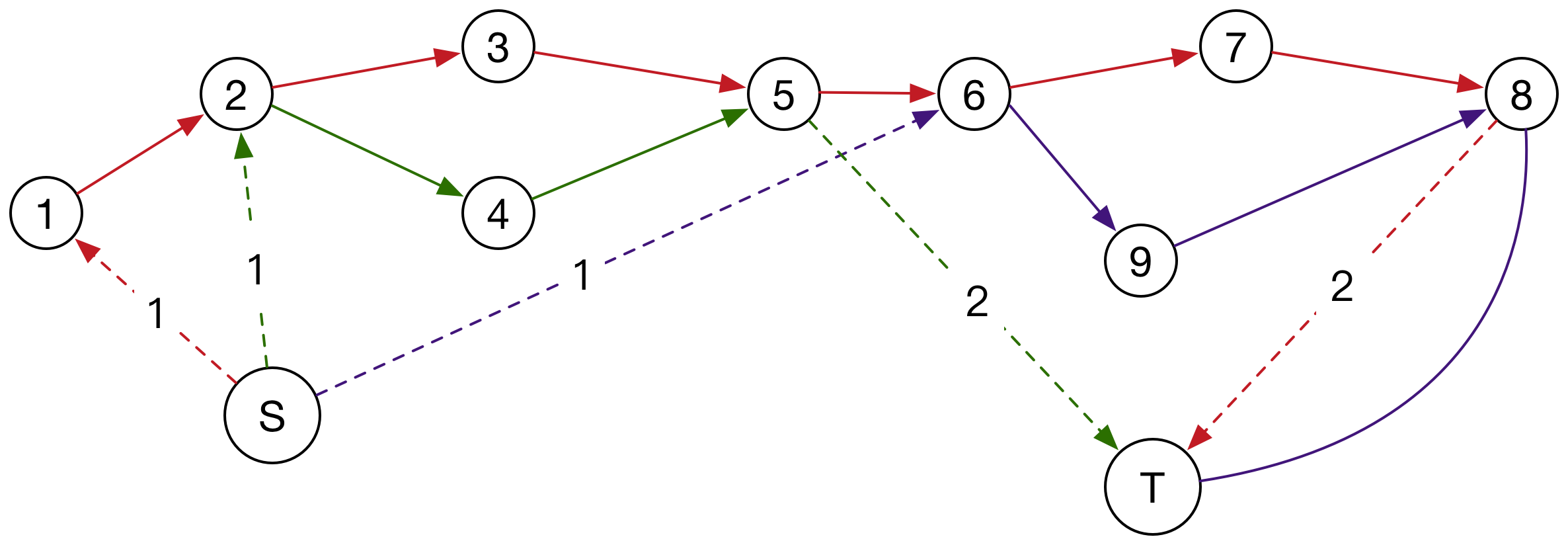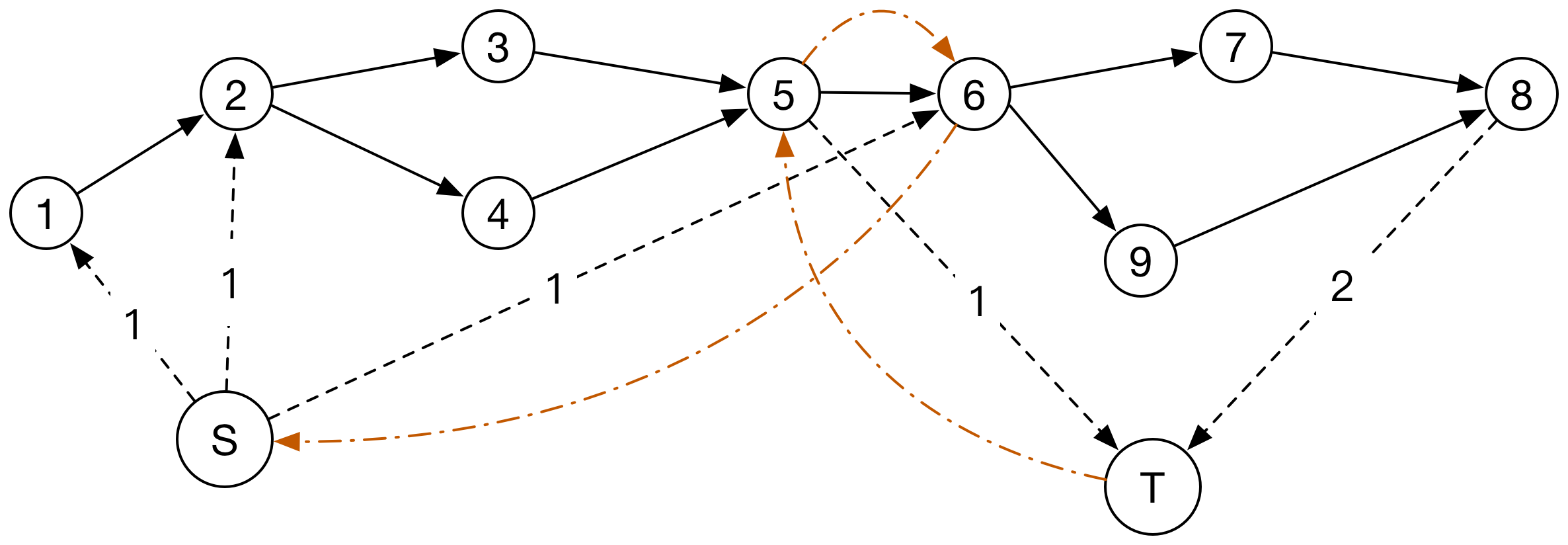# UVa 1440 - Inspection

1. 添加超级源点、汇点 ， 并找到一个可行流（满足下界的流量图）
2. 返回尽量多的流 ， 并且保持 满足下界 这个条件
3. 输出一组方案

• 怎么找到一个可行流呢？ 可以按照白书上面的思路 ， 重置路径 ， 然后求一组可行解 。 但基于这个问题的特殊性（DAG$DAG$） ，我们可以构造一个刚好满足下界的可行流。

• 对于每个点 ， 计算入度和出度。 对于每一个点 ， 如果出度>入度 ， 那么我们从超级源点S向这个点连一条边 ， 流量为这个点的出度－入度。这相当于把这个点当作起点 ， 开始一些新的航行。 相似的 ， 如果入度>出度 ， 那么我们从这个点向超级汇点连一条边 ， 流量为入度－出度。

• 图示如下：• 那么 ， 怎么返回尽量多的流量呢？ 首先让我们看看这个图怎么返回流量的:• 显然 ， 这个图只需要两次航行就可以了 ， 但这里的流量为3 ， 所以有一个流量需要从T经过反向边 ， 回到S。 如图 ， T>5>6>S$T -> 5 -> 6 -> S$就是这样的一条路径。
• 其实在修改后的图中 ， 另一个航行是沿着：
S>2>4>5>6>9>8>T$S -> 2 -> 4 -> 5 -> 6 -> 9 -> 8 -> T$

• 这就启发我们 ， 在不违反下界的前提下 ， 找一个从T$T$S$S$的最大流就OK了。
UP： 那如何不违反下界呢 ， 其实我们在建图的时候耍一点小把戏 ， 把有下界的边的反向边容量设为0就可以啦！

#include <iostream>
#include <cstdio>
#include <cstdlib>
#include <cstring>
#include <cmath>
#include <string>
#include <vector>
#include <deque>
#include <stack>
#include <algorithm>
#include <queue>

using namespace std;
const int maxn = 150;
const int INF = 0x3f3f3f3f;
struct edge { int t , c , re , v; edge(int t = 0 , int c =0  , int re = 0 , int v = 0):t(t),c(c),re(re),v(v){} };

int n;
vector<edge> g[maxn];
int d[maxn];

void addEdge(int a , int b , int c , int modi = 0)
{
g[a].push_back(edge(b , c , g[b].size() , 1));
g[b].push_back(edge(a , 0 , g[a].size() - 1));

if(modi)
{
g[a].back().c-=modi;
g[b].back().c+=modi;
}
}

int level[maxn];
int iter[maxn];

bool bfs(int s , int t)
{
memset(level, INF, sizeof(level));
queue<int> q;
q.push(s);
level[s] = 0;

while(!q.empty())
{
int now = q.front();
q.pop();

for(int i=0;i<g[now].size();i++)
{
edge& e = g[now][i];
if(level[e.t]==INF && e.c>0)
{
level[e.t] = level[now]+1;
q.push(e.t);
}
}
}
return level[t]!=INF;
}

int dfs(int s , int t , int flow)
{
if(s==t || !flow) return flow;

for(int& i = iter[s];i<g[s].size();i++)
{
edge& e = g[s][i];
if(level[e.t]!=level[s]+1) continue;

int f = dfs(e.t, t, min(flow, e.c));
if(f>0)
{
e.c-=f;
g[e.t][e.re].c+=f;
return f;
}
}
return 0;
}

int MaxFlow(int s , int t)
{
int res = 0;
while(bfs(s, t))
{
memset(iter, 0, sizeof(iter));
int flow;
while((flow = dfs(s, t, INF))>0) res+= flow;
}
return res;
}

bool extend(int u , int s , int t)
{
if(u==t) return true;
for(int i=0;i<g[u].size();i++)
{
edge& e = g[u][i];
if(!g[e.t][e.re].c || !e.v) continue;
g[e.t][e.re].c--;
if(e.t!=t) cout<<(u==s?"":" ")<<e.t;
return extend(e.t, s, t);
}
return false;
}

int main(int argc, char *argv[]) {

while(cin>>n && n)
{
memset(d, 0, sizeof(d));
for(int i=0;i<=n+1;i++) g[i].clear();

for(int i=1;i<=n;i++)
{
int num;
cin>>num;

while(num--)
{
int x;
cin>>x;
d[x]++;
d[i]--;
}
}
int S = 0 , T = n+1 , flow = 0;
for(int i=1;i<=n;i++)
if(d[i]>0)
addEdge(i, T, INF , d[i]) , flow+=d[i];
else if(d[i]<0)

flow-= MaxFlow(T, S);

for(int i=1;i<=n;i++) for(int j=0;j<g[i].size();j++) if(g[i][j].v && g[i][j].t!=S && g[i][j].t!=T)
{
edge& e = g[i][j];
g[e.t][e.re].c++;
}

cout<<flow<<endl;
while(extend(S, S, T)) cout<<endl;

}

return 0;
}

01-113641

#### Eclipse中jsp页面中js脚本报错this inspection checks that the script tag content is valid XML.

06-191万+

#### IntelliJ IDEA关闭“Found duplicate code in”提示10-221379

#### UVA1440 有下界的最小流

02-211万+

#### idea显示：Inspection info:Detects duplicates in source code

05-02152

#### uva 1440

07-211777

#### UVa 1440 只有下界的最小流

10-20888

#### Uva-1440-Inspection (只有下界的网络最小流)

03-061109

#### （intermediate） 带下界的最小流 UVA 1440 - Inspection

11-30885

#### UVa 11014 - Make a Crystal

07-14871

#### Uva-1440-Inspection©️2020 CSDN 皮肤主题: 大白 设计师: CSDN官方博客点击重新获取扫码支付1.余额是钱包充值的虚拟货币，按照1:1的比例进行支付金额的抵扣。
2.余额无法直接购买下载，可以购买VIP、C币套餐、付费专栏及课程。余额充值